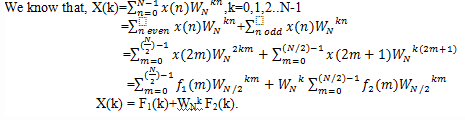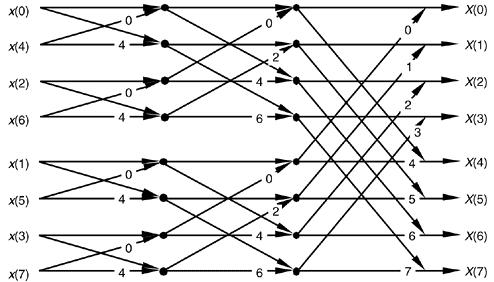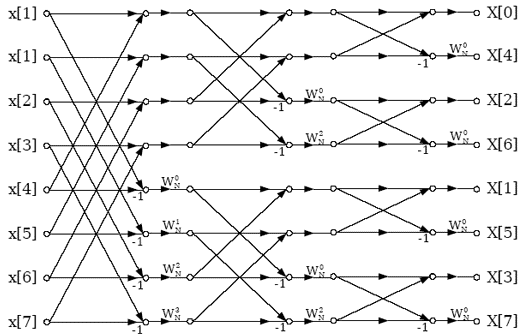Test: DFT Algorithm Computation - 2

# Test: DFT Algorithm Computation - 2

Test Description

## 10 Questions MCQ Test Signals and Systems | Test: DFT Algorithm Computation - 2

Test: DFT Algorithm Computation - 2 for Electrical Engineering (EE) 2023 is part of Signals and Systems preparation. The Test: DFT Algorithm Computation - 2 questions and answers have been prepared according to the Electrical Engineering (EE) exam syllabus.The Test: DFT Algorithm Computation - 2 MCQs are made for Electrical Engineering (EE) 2023 Exam. Find important definitions, questions, notes, meanings, examples, exercises, MCQs and online tests for Test: DFT Algorithm Computation - 2 below.
Solutions of Test: DFT Algorithm Computation - 2 questions in English are available as part of our Signals and Systems for Electrical Engineering (EE) & Test: DFT Algorithm Computation - 2 solutions in Hindi for Signals and Systems course. Download more important topics, notes, lectures and mock test series for Electrical Engineering (EE) Exam by signing up for free. Attempt Test: DFT Algorithm Computation - 2 | 10 questions in 10 minutes | Mock test for Electrical Engineering (EE) preparation | Free important questions MCQ to study Signals and Systems for Electrical Engineering (EE) Exam | Download free PDF with solutions
 1 Crore+ students have signed up on EduRev. Have you?
Test: DFT Algorithm Computation - 2 - Question 1

### If we split the N point data sequence into two N/2 point data sequences f1(n) and f2(n) corresponding to the even numbered and odd numbered samples of x(n), then such an FFT algorithm is known as decimation-in-time algorithm.

Detailed Solution for Test: DFT Algorithm Computation - 2 - Question 1

Explanation: Let us consider the computation of the N=2v point DFT by the divide and conquer approach. We select M=N/2 and L=2. This selection results in a split of N point data sequence into two N/2 point data sequences f1(n) and f2(n) corresponding to the even numbered and odd numbered samples of x(n), respectively, that is
f1(n)=x(2n)
f2(n)=x(2n+1) ,n=0,1,2…N/2-1
Thus f1(n) and f2(n) are obtained by decimating x(n) by a factor of
2, and hence the resulting FFT algorithm is called a decimation-in-time algorithm.

Test: DFT Algorithm Computation - 2 - Question 2

### If we split the N point data sequence into two N/2 point data sequences f1(n) and f2(n) corresponding to the even numbered and odd numbered samples of x(n) and F1(k) and F2(k) are the N/2 point DFTs of f1(k) and f2(k) respectively, then what is the N/2 point DFT X(k) of x(n)?

Detailed Solution for Test: DFT Algorithm Computation - 2 - Question 2

Explanation: From the question, it is given that
f1(n)=x(2n)
f2(n)=x(2n+1) ,n=0,1,2…N/2-1Test: DFT Algorithm Computation - 2 - Question 3

### If X(k) is the N/2 point DFT of the sequence x(n), then what is the value of X(k+N/2)?

Detailed Solution for Test: DFT Algorithm Computation - 2 - Question 3

Explanation: We know that, X(k) = F1(k)+WNk F2(k)
We know that F1(k) and F2(k) are periodic, with period N/2, we have F1(k+N/2)= F1(k) and F2(k+N/2)= F2(k). In addition, the factor WNk+N/2= -WNk.
Thus we get, X(k+N/2)= F1(k)- WNk F2(k).

Test: DFT Algorithm Computation - 2 - Question 4

How many complex multiplications are required to compute X(k)?

Detailed Solution for Test: DFT Algorithm Computation - 2 - Question 4

Explanation: We observe that the direct computation of F1(k) requires (N/2)2 complex multiplications. The same applies to the computation of F2(k). Furthermore, there are N/2 additional complex multiplications required to compute WNk. Hence it requires N(N+1)/2 complex multiplications to compute X(k).

Test: DFT Algorithm Computation - 2 - Question 5

The total number of complex multiplications required to compute N point DFT by radix-2 FFT is:

Detailed Solution for Test: DFT Algorithm Computation - 2 - Question 5

Explanation: The decimation of the data sequence should be repeated again and again until the resulting sequences are reduced to one point sequences. For N=2v, this decimation can be performed v=log2N times. Thus the total number of complex multiplications is reduced to (N/2)log2N.

Test: DFT Algorithm Computation - 2 - Question 6

The total number of complex additions required to compute N point DFT by radix-2 FFT is:

Detailed Solution for Test: DFT Algorithm Computation - 2 - Question 6

Explanation: The decimation of the data sequence should be repeated again and again until the resulting sequences are reduced to one point sequences. For N=2v, this decimation can be performed v=log2N times. Thus the total number of complex additions is reduced to Nlog2N.

Test: DFT Algorithm Computation - 2 - Question 7

The following butterfly diagram is used in the computation of:Detailed Solution for Test: DFT Algorithm Computation - 2 - Question 7

Explanation: The above given diagram is the basic butterfly computation in the decimation-in-time FFT algorithm.

Test: DFT Algorithm Computation - 2 - Question 8

For a decimation-in-time FFT algorithm, which of the following is true?

Detailed Solution for Test: DFT Algorithm Computation - 2 - Question 8

Explanation: In decimation-in-time FFT algorithm, the input is taken in bit reversal order and the output is obtained in the order.

Test: DFT Algorithm Computation - 2 - Question 9

The following butterfly diagram is used in the computation of:Detailed Solution for Test: DFT Algorithm Computation - 2 - Question 9

Explanation: The above given diagram is the basic butterfly computation in the decimation-in-frequency FFT algorithm.

Test: DFT Algorithm Computation - 2 - Question 10

For a decimation-in-time FFT algorithm, which of the following is true?

Detailed Solution for Test: DFT Algorithm Computation - 2 - Question 10

Explanation: In decimation-in-frequency FFT algorithm, the input is taken in order and the output is obtained in the bit reversal order.

## Signals and Systems

32 videos|76 docs|63 tests
 Use Code STAYHOME200 and get INR 200 additional OFF Use Coupon Code
Information about Test: DFT Algorithm Computation - 2 Page
In this test you can find the Exam questions for Test: DFT Algorithm Computation - 2 solved & explained in the simplest way possible. Besides giving Questions and answers for Test: DFT Algorithm Computation - 2, EduRev gives you an ample number of Online tests for practice

## Signals and Systems

32 videos|76 docs|63 tests